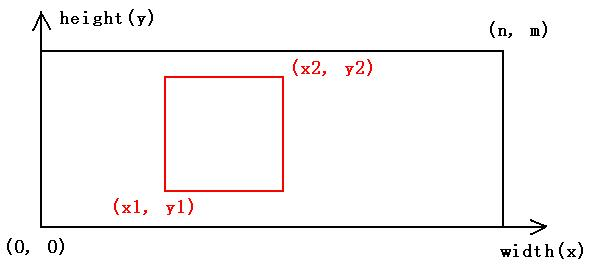# Treasure Map（ZOJ - 3209）

Your boss once had got many copies of a treasure map. Unfortunately, all the copies are now broken to many rectangular pieces, and what make it worse, he has lost some of the pieces. Luckily, it is possible to figure out the position of each piece in the original map. Now the boss asks you, the talent programmer, to make a complete treasure map with these pieces. You need to make only one complete map and it is not necessary to use all the pieces. But remember, pieces are not allowed to overlap with each other (See sample 2).

Input

The first line of the input contains an integer T (T <= 500), indicating the number of cases.

For each case, the first line contains three integers n m p (1 <= n, m <= 30, 1 <= p <= 500), the width and the height of the map, and the number of pieces. Then p lines follow, each consists of four integers x1 y1 x2 y2 (0 <= x1 < x2 <= n, 0 <= y1 < y2 <= m), where (x1, y1) is the coordinate of the lower-left corner of the rectangular piece, and (x2, y2) is the coordinate of the upper-right corner in the original map.

Cases are separated by one blank line.Output

If you can make a complete map with these pieces, output the least number of pieces you need to achieve this. If it is impossible to make one complete map, just output -1.
Sample Input

3
5 5 1
0 0 5 5

5 5 2
0 0 3 5
2 0 5 5

30 30 5
0 0 30 10
0 10 30 20
0 20 30 30
0 0 15 30
15 0 30 30
Sample Output

1
-1
2
Hint

For sample 1, the only piece is a complete map.

For sample 2, the two pieces may overlap with each other, so you can not make a complete treasure map.

For sample 3, you can make a map by either use the first 3 pieces or the last 2 pieces, and the latter approach one needs less pieces.

#include <iostream>
#include <cstdio>
#include <cstring>

using namespace std;

const int maxnode = 500010;
const int MaxM = 1010;
const int MaxN = 510;
struct DLX
{
int n,m,siz;
int U[maxnode],D[maxnode],R[maxnode],L[maxnode],Row[maxnode],Col[maxnode];
int H[MaxN],S[MaxM];
int ansed;
void init(int _n,int _m)
{
n = _n;
m = _m;
for(int i = 0;i <= m;i++)
{
S[i] = 0;
U[i] = D[i] = i;
L[i] = i-1;
R[i] = i+1;
}
R[m] = 0; L = m;
siz = m;
for(int i = 1;i <= n;i++)
H[i] = -1;
}
{
++S[Col[++siz]=c];
Row[siz] = r;
D[siz] = D[c];
U[D[c]] = siz;
U[siz] = c;
D[c] = siz;
if(H[r] < 0)H[r] = L[siz] = R[siz] = siz;
else
{
R[siz] = R[H[r]];
L[R[H[r]]] = siz;
L[siz] = H[r];
R[H[r]] = siz;
}
}
void del(int c)
{
L[R[c]] = L[c]; R[L[c]] = R[c];
for(int i = D[c];i != c;i = D[i])
for(int j = R[i];j != i;j = R[j])
{
U[D[j]] = U[j];
D[U[j]] = D[j];
--S[Col[j]];
}
}
void recover(int c)
{
for(int i = U[c];i != c;i = U[i])
for(int j = L[i];j != i;j = L[j])
++S[Col[U[D[j]]=D[U[j]]=j]];
L[R[c]] = R[L[c]] = c;
}

void Dancing(int d)
{
if(ansed != -1 && ansed <= d)return;
if(R == 0)
{
if(ansed == -1)ansed = d;
else if(d < ansed)ansed = d;
return;
}
int c = R;
for(int i = R;i != 0;i = R[i])
if(S[i] < S[c])
c = i;
del(c);
for(int i = D[c];i != c;i = D[i])
{
for(int j = R[i];j != i;j = R[j])del(Col[j]);
Dancing(d+1);
for(int j = L[i];j != i;j = L[j])recover(Col[j]);
}
recover(c);
}
}dlx;

int main()
{
int t;
int n,m,p;
cin>>t;
while(t--)
{
cin>>n>>m>>p;
dlx.init(p,n*m);
int x1,y1,x2,y2;
for(int k = 1;k <= p;k++)
{
cin>>x1>>y1>>x2>>y2;
for(int i = x1+1;i <= x2;i++)
for(int j = y1+1;j <= y2;j++)
}
dlx.ansed = -1;
dlx.Dancing(0);
cout<<dlx.ansed<<endl;
}
return 0;
}©️2019 CSDN 皮肤主题: 技术黑板 设计师: CSDN官方博客